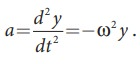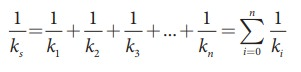Home | | Physics 11th std | Summary - Physics: Oscillations

# Summary - Physics: Oscillations

Physics : Oscillations - Summary - Physics: Oscillations

SUMMARY

When an object or a particle moves back and forth repeatedly about a reference point for some duration of time it is said to have Oscillatory (or vibratory) motion.

For a SHM, the acceleration or force on the particle is directly proportional to its displacement from a fixed point and always directed towards that fixed point. The force is

Fx = − k x

where k is a constant whose dimension is force per unit length, called as force constant.

In Simple harmonic motion, the displacement, y = A sin ωt.

In Simple harmonic motion, the velocity, v = A ω cos ωt = ω√[A2-y2]

In Simple harmonic motion, the acceleration, a =The time period is defined as the time taken by a particle to complete one oscillation. It is usually denoted by T. Time period T 2π/ω

The number of oscillations produced by the particle per second is called frequency. It is denoted by f. SI unit for frequency is S−1 or hertz (In symbol, Hz). Mathematically, frequency is related to time period by f =1/T.

The frequency of the angular harmonic motion isFor n springs connected in series, the effective spring constant in series isFor n springs connected in parallel, the effective spring constant isThe time period for U-tube oscillation is TFor a conservative system in one dimension, the force field can be derived from a scalar potential energy:In a simple harmonic motion, potential energy is U ( x).In a simple harmonic motion, kinetic energy is KETotal energy for a simple harmonic motion is.

Types of oscillations – Free oscillations, Damped oscillations, Maintained oscillations and Forced oscillations.

Resonance is a special case of forced oscillations.

Study Material, Lecturing Notes, Assignment, Reference, Wiki description explanation, brief detail
11th Physics : UNIT 10 : Oscillations : Summary - Physics: Oscillations |